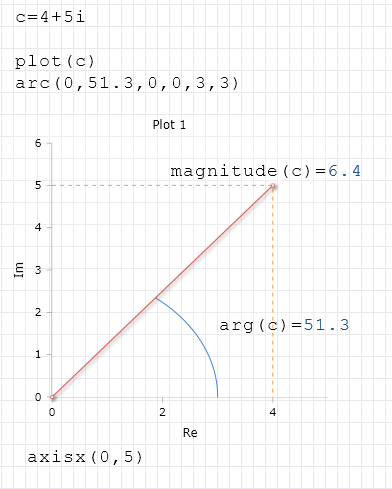# FromPolar Function

Compute a complex number based on its polar coordinates

### Description

The function $$FromPolar$$ computes a complex number from the polar coordinates of a point in the coordinate system.

Since there are multiple representations of a point at a complex level, the function's return value is normalized to a positive number, and the angle is normalized to a value in the range of $$-Pi$$ to $$Pi$$. As a result, the values of the angle and magnitude correspond to the resulting complex number may not match the original values of the angle parameter.

### Syntax

FromPolar (magnitude, angle)

### Example

FromPolar(6.4,51.31)= 4+5i